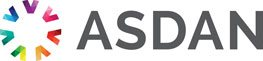# Lifeskills Challenge

## Working with fractions

### Challenge reference: 3109

Notional learning hours 30 1 The learner will develop skills and confidence in calculating with fractions. Please log in to see the rest of this challenge

### Learning outcomes

What the learner needs to know, understand or be able to do

The learner will:

1. Be able to order and compare basic fractions.

2. Be able to order and compare improper fractions and mixed numbers.

### Assessment criteria

What the learner need to demonstrate in order to meet the learning outcome

The learner can:

• Compare basic fractions and identify:
•  smallest
•  largest
•  equivalence
•  Simplify basic fractions into an equivalent form, working in appropriate denominators
• Change improper fractions into mixed numbers
• Change mixed numbers into improper fractions
• Compare improper fractions and identify:
• smallest
• larges